# Flood Fill

Flood fill is a method that is surprisingly useful in a large number of different situations and keeps finding me wherever I go. When I was completing my PhD, I had an idea to track superfluid vortices by using flood fill as a way to help mask out unnecessary features of the simulation. When I was making a terminal game, I thought of creating an animation that was just flood fill in disguise. When I decided to play minesweeper or Go with my girlfriend, flood fill was used in both!

Flood fill is probably most commonly known as the "Bucket Fill" application in most art programs . It's usually indicated by an icon that looks like a bucket and is known to fill in any enclosed area, as shown below: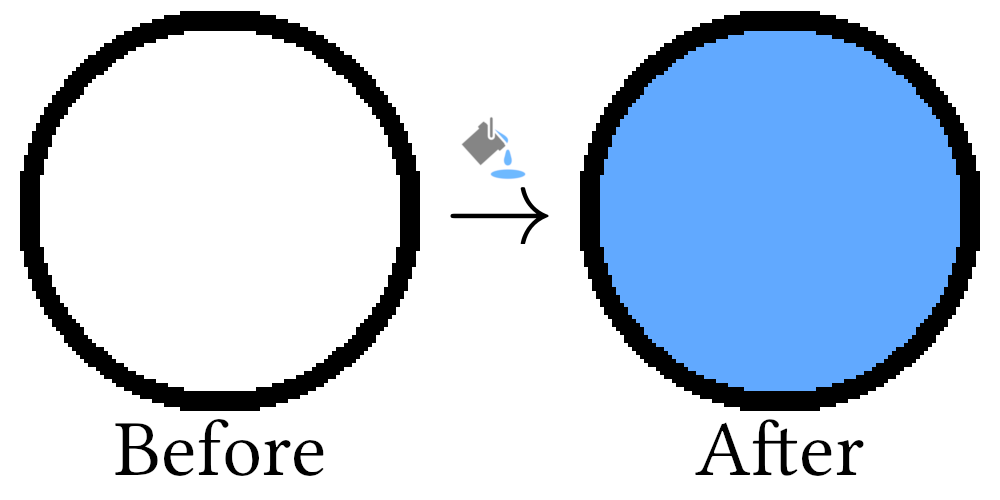Because flood fill is incredibly common, there are a large number of variations to the method, some of which are more optimal than others. For this chapter, we will cover the basics: how to fill a domain in a quick and dirty way. In subsequent chapters, we will continue our journey by creating more and more efficient flood fill methods, including scanline-based and fixed memory methods .

I have decided to split the chapter up for a few important reasons:

1. I did not want to flood the Algorithm Archive with flood fill methods all at the same time. I feel it's worth letting each chapter sit for a bit while we savor it's unique flavor.
2. Many users are implementing versions of each algorithm in their own languages and it is difficult to review and submit code for chapters with a lot of code chunks. Several sub-chapters with less code is easier for everyone.
3. I am kinda under a time-constraint right now and wanted to make sure we regularly get content into the Algorithm Archive.

So, without further a-do, let's hop right into it!

## What does flood fill do?

Flood fill is essentially composed of 2 parts:

1. Determining the extents of the domain to fill
2. Walking through all elements within a domain and changing some property

For the purposes of this chapter, we will be using a set of floating-point values that range from 0 to 1 instead of a color-space like RGB. Though bucket fill is always used in art programs in some sort of color space, flood fill is more general and can be used in a space with any type of element. As such, it makes sense to use a simpler element type so we can better understand the method.

So how do we go about finding the extents of the domain to fill?

Here, a domain will be defined as any connected set of elements in an -dimensional space whose values do not vary beyond a predefined threshold. As an example, if we take a circle embedded into a 2-dimensional grid, we have 3 separate domains:

1. Inside the circle where all elements are 0.
2. The circle, itself, where the elements are set to 0.75.
3. Outside the circle where all elements are similarly 0.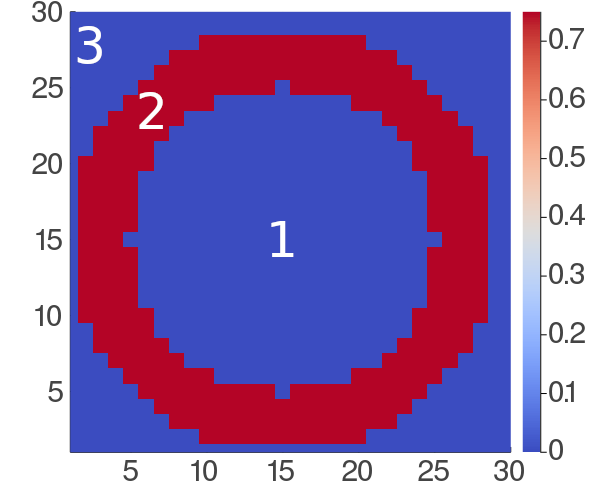Though there are some more complicated ways to determine the extents of the domain, we will not focus on this aspect of the flood fill method for the remainder of this chapter and instead leave it for subsequent chapters. So now we will focus on the process of walking through each element in the domain and changing some property.

## Domain traversal

As before, the simplest example to work with is that of an image, where each element in our domain is a single pixel. Here, we can connect each pixel to all other pixels in its vicinity, like so: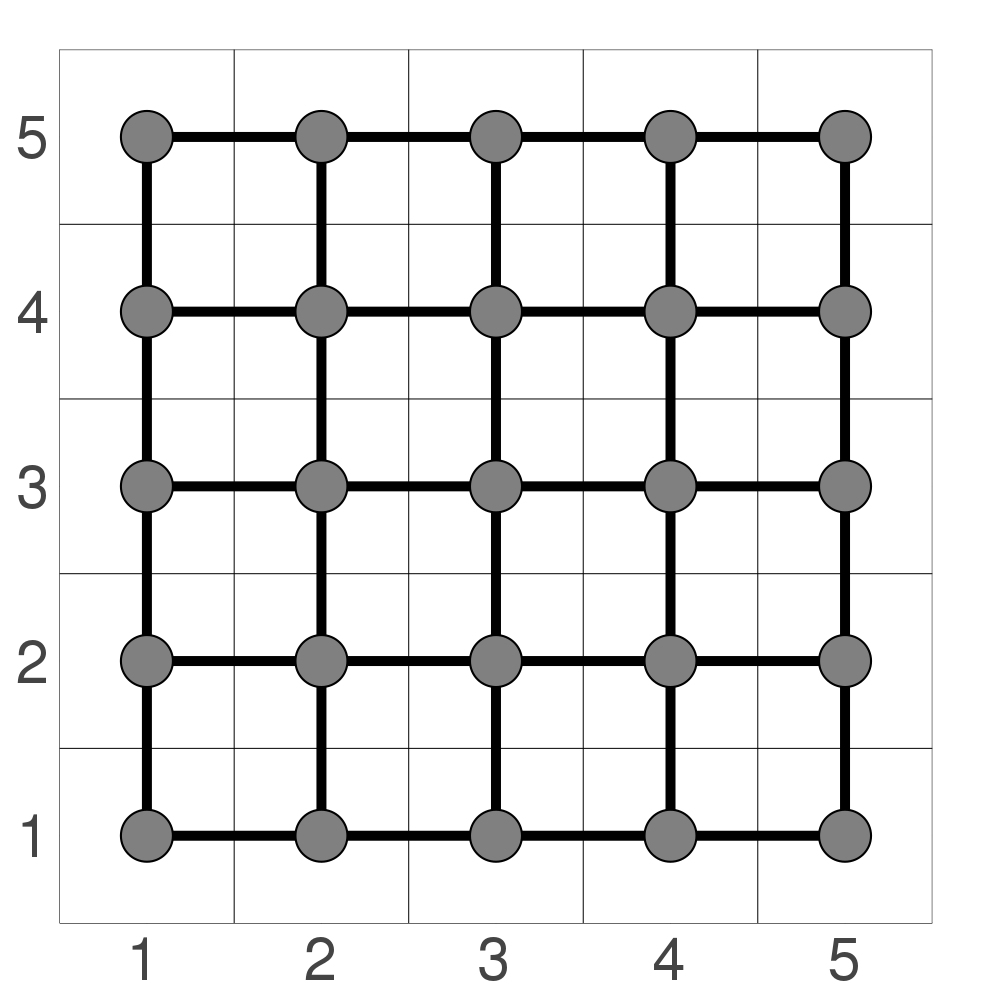In this image, a border is shown between each individual pixel and a grid is superimposed to show how each pixel is connected to its neighbors. This means that each element has 4 neighbors: north, south, east, and west. We could also include northeast, southeast, southwest, and northwest if we wanted to do an 8-way fill, but we will restrict the discussion to the 4-way fill for now, as the method is essentially the same and slightly easier to understand with fewer elements to worry about.

By connecting each pixel to its neighbors in this way, the flood fill operation becomes a process of graph traversal, not too dissimilar from the tree traversal methods described before. This means that after selecting our initial location, we can then traverse through all elements in either a depth-first or breadth-first fashion. We will be covering the following this chapter:

1. Finding all neighbors
2. Depth-first node traversal
3. Breadth-first node traversal and small-scale optimizations

So let's start by discussing how we might go about finding the neighbors to fill.

### Finding all neighbors

The first step of this method is to query the location of all possible neighbors. At first glance, this seems rather straightforward. One simply needs to look up, down, left, and right of the current location and add those elements to the list of neighbors if they are:

1. On the canvas
2. Have a value close enough to the old value we would like to replace

In code, this might look like this:

function find_neighbors(canvas, loc::CartesianIndex, old_val, new_val)

# Finding north, south, east, west neighbors
possible_neighbors = [loc + CartesianIndex(0, 1),
loc + CartesianIndex(1, 0),
loc + CartesianIndex(0, -1),
loc + CartesianIndex(-1, 0)]

# Exclusing neighbors that should not be colored
neighbors =  []
for possible_neighbor in possible_neighbors
if inbounds(size(canvas), possible_neighbor) &&
canvas[possible_neighbor] == old_val
push!(neighbors, possible_neighbor)
end
end

return neighbors
end

int find_neighbors(struct canvas c, struct point p, int old_val,
struct point *neighbors) {
int cnt = 0;
struct point points = {
{p.x, p.y + 1},
{p.x + 1, p.y},
{p.x, p.y - 1},
{p.x - 1, p.y}
};

for (int i = 0; i < 4; ++i) {
if (inbounds(points[i], c) &&
c.data[points[i].x + c.max_x * points[i].y] == old_val) {
neighbors[cnt++] = points[i];
}
}

return cnt;
}

auto find_neighbors(
std::vector<std::vector<float>> const& grid,
CartesianIndex loc,
float old_value,
float /* new_value */) {

const std::vector<CartesianIndex> possible_neighbors{
{loc, loc + 1},
{loc + 1, loc},
{loc, loc - 1},
{loc - 1, loc}};

std::vector<CartesianIndex> neighbors;

for (auto const& possible_neighbor : possible_neighbors) {
const auto size = CartesianIndex{
static_cast<int>(grid.size()), static_cast<int>(grid.size())};
const auto x = static_cast<std::size_t>(possible_neighbor);
const auto y = static_cast<std::size_t>(possible_neighbor);
if (inbounds(size, possible_neighbor) && grid[x][y] == old_value) {
neighbors.push_back(possible_neighbor);
}
}

return neighbors;
}

def find_neighbors(canvas, p, old_val, new_val):
# north, south, east, west neighbors
possible_neighbors = [
Point(p.x, p.y+1),
Point(p.x+1, p.y),
Point(p.x-1, p.y),
Point(p.x, p.y-1)
]

# exclude the neighbors that go out of bounds and should not be colored
neighbors = []
for possible_neighbor in possible_neighbors:
if inbounds(canvas.shape, possible_neighbor):
if canvas[possible_neighbor] == old_val:
neighbors.append(possible_neighbor)
return neighbors

def find_neighbours(canvas, location is Point, old_value):
possible_neighbours = ((Point(0, 1), Point(1, 0), Point(0, -1), Point(-1, 0))
|> map$(location.__add__)) yield from possible_neighbours |> filter$(x -> (inbounds(canvas.shape, x)
and canvas[x] == old_value))


This code is set up to return a vector of elements to then use for subsequent sections.

### Depth-first node traversal

Now that we have the ability to find all neighboring elements, we can proceed to traverse through those nodes in the most straightforward way: recursion.

In code, it might look like this:

function recursive_fill!(canvas, loc::CartesianIndex, old_val, new_val)

if (old_val == new_val)
return
end

canvas[loc] = new_val

possible_neighbors = find_neighbors(canvas, loc, old_val, new_val)
for possible_neighbor in possible_neighbors
recursive_fill!(canvas, possible_neighbor, old_val, new_val)
end
end

void recursive_fill(struct canvas c, struct point p, int old_val,
int new_val) {

if (old_val == new_val) {
return;
}

c.data[p.x + c.max_x * p.y] = new_val;

struct point neighbors;
int cnt = find_neighbors(c, p, old_val, neighbors);

for (int i = 0; i < cnt; ++i) {
recursive_fill(c, neighbors[i], old_val, new_val);
}
}

void recursive_fill(
std::vector<std::vector<float>>& grid,
CartesianIndex loc,
float old_value,
float new_value) {
if (old_value == new_value) {
return;
}

const auto x = static_cast<std::size_t>(loc);
const auto y = static_cast<std::size_t>(loc);

grid[x][y] = new_value;

const auto possible_neighbors = find_neighbors(grid, loc, old_value, new_value);
for (auto const& possible_neighbor : possible_neighbors) {
recursive_fill(grid, possible_neighbor, old_value, new_value);
}
}

def recursive_fill(canvas, p, old_val, new_val):
if old_val == new_val:
return

canvas[p] = new_val

neighbors = find_neighbors(canvas, p, old_val, new_val)
for neighbor in neighbors:
recursive_fill(canvas, neighbor, old_val, new_val)

def recursive_fill(canvas, location is Point, old_value, new_value):
if new_value == old_value or not inbounds(canvas.shape, location):
return

canvas[location] = new_value
# consume is important here, because otherwise, the recursive function is not called again
consume(
find_neighbours(canvas, location, old_value)
|> map$(recursive_fill$(canvas, ?, old_value, new_value))
)


The above code continues recursing through available neighbors as long as neighbors exist, and this should work so long as we are adding the correct set of neighbors.

Additionally, it is possible to do the same type of traversal by managing a stack, like so:

function stack_fill!(canvas, loc::CartesianIndex, old_val, new_val)
if new_val == old_val
return
end

s = Stack{CartesianIndex}()
push!(s, loc)

while length(s) > 0
current_loc = pop!(s)
if canvas[current_loc] == old_val
canvas[current_loc] = new_val
possible_neighbors = find_neighbors(canvas, current_loc,
old_val, new_val)
for neighbor in possible_neighbors
push!(s,neighbor)
end
end

end
end

void stack_fill(struct canvas c, struct point p, int old_val, int new_val) {
if (old_val == new_val) {
return;
}

struct stack stk = get_stack();
stack_push(&stk, p);

while (!stack_empty(stk)) {
struct point cur_loc = stack_pop(&stk);
if (c.data[cur_loc.x + c.max_x * cur_loc.y] == old_val) {
c.data[cur_loc.x + c.max_x * cur_loc.y] = new_val;

struct point neighbors;
int cnt = find_neighbors(c, cur_loc, old_val, neighbors);

for (int i = 0; i < cnt; ++i) {
stack_push(&stk, neighbors[i]);
}
}
}

free_stack(stk);
}

void stack_fill(
std::vector<std::vector<float>>& grid,
CartesianIndex loc,
float old_value,
float new_value) {
if (old_value == new_value) {
return;
}

auto s = std::stack<CartesianIndex>{};
s.push(loc);

while (s.size() > 0) {
const auto current_loc = s.top();
s.pop();

const auto x = static_cast<std::size_t>(current_loc);
const auto y = static_cast<std::size_t>(current_loc);

if (grid[x][y] == old_value) {
grid[x][y] = new_value;
const auto possible_neighbors =
find_neighbors(grid, current_loc, old_value, new_value);
for (auto const& neighbor : possible_neighbors) {
s.push(neighbor);
}
}
}
}

def stack_fill(canvas, p, old_val, new_val):
if old_val == new_val:
return

stack = [p]

while stack:
cur_loc = stack.pop()
canvas[cur_loc] = new_val
stack += find_neighbors(canvas, cur_loc, old_val, new_val)

def stack_fill(canvas, location is Point, old_value, new_value):
if new_value == old_value or not inbounds(canvas.shape, location):
return

stack = [location]

while stack:
current_location = stack.pop()
if canvas[current_location] == old_value:
canvas[current_location] = new_value
stack.extend(find_neighbours(canvas, current_location, old_value))


This is ultimately the same method of traversal as before; however, because we are managing our own data structure, there are a few distinct differences:

1. The manually managed stack could be slightly slower and potentially more memory-intensive
2. It is easy to reach the maximum recursion depth on certain hardware with the recursive method, so it is best to use the stack-based implementation in those cases.

If we were to use either of these methods to fill a circle embedded in a two dimensional domain, we would see the following

Here, we see that these methods will traverse through one direction first before filling from there. This is potentially the easiest method to write, but it is not the most intuitive fill pattern. I suspect that if someone was asked to fill the contents of the circle on their own, they would fill it more evenly from the center, like so:

This is simply another traversal strategy known as breadth-first traversal and comes with its own set of caveats. We will discuss this further in the next subsection

### Breadth-first node traversal and small-scale optimizations

Breadth-first node traversal is as simple as switching the stack in the depth-first strategy with a queue. The code would look something like this:

function queue_fill!(canvas, loc::CartesianIndex, old_val, new_val)
if new_val == old_val
return
end

q = Queue{CartesianIndex}()
enqueue!(q, loc)

# Coloring the initial location
canvas[loc] = new_val

while length(q) > 0
current_loc = dequeue!(q)

possible_neighbors = find_neighbors(canvas, current_loc,
old_val, new_val)

# Coloring as we are enqueuing neighbors
for neighbor in possible_neighbors
canvas[neighbor] = new_val
enqueue!(q,neighbor)
end

end
end

void queue_fill(struct canvas c, struct point p, int old_val, int new_val) {
if (old_val == new_val) {
return;
}

struct queue q = get_queue(sizeof(struct point *));
enqueue(&q, p);

while (!queue_empty(q)) {
struct point cur_loc = dequeue(&q);
if (c.data[cur_loc.x + c.max_x * cur_loc.y] == old_val) {
c.data[cur_loc.x + c.max_x * cur_loc.y] = new_val;

struct point neighbors;
int cnt = find_neighbors(c, cur_loc, old_val, neighbors);

for (int i = 0; i < cnt; ++i) {
enqueue(&q, neighbors[i]);
}
}
}

free_queue(q);
}

void queue_fill(
std::vector<std::vector<float>>& grid,
CartesianIndex loc,
float old_value,
float new_value) {
if (old_value == new_value) {
return;
}

auto q = std::queue<CartesianIndex>{};
q.push(loc);
const auto x = static_cast<std::size_t>(loc);
const auto y = static_cast<std::size_t>(loc);
grid[x][y] = new_value;

while (q.size() > 0) {
const auto current_loc = q.front();
q.pop();
const auto possible_neighbors =
find_neighbors(grid, current_loc, old_value, new_value);
for (auto const& neighbor : possible_neighbors) {
const auto neighbor_x = static_cast<std::size_t>(neighbor);
const auto neighbor_y = static_cast<std::size_t>(neighbor);
grid[neighbor_x][neighbor_y] = new_value;
q.push(neighbor);
}
}
}

def queue_fill(canvas, p, old_val, new_val):
if old_val == new_val:
return

q = Queue()
q.put(p)

canvas[p] = new_val

while not q.empty():
cur_loc = q.get()
neighbors = find_neighbors(canvas, cur_loc, old_val, new_val)

for neighbor in neighbors:
canvas[neighbor] = new_val
q.put(neighbor)

def queue_fill(canvas, location is Point, old_value, new_value):
if new_value == old_value or not inbounds(canvas.shape, location):
return

queue = deque()
queue.append(location)

canvas[location] = new_value

while queue:
current_location = queue.popleft()
for neighbour in find_neighbours(canvas, current_location, old_value):
canvas[neighbour] = new_value
queue.append(neighbour)


Now, there is a small trick in this code that must be considered to make sure it runs optimally. Namely, the nodes must be colored when they are being enqueued, not when visiting the node. At least for me, it was not immediately obvious why this would be the case, but let me try to explain.

Let's imagine that we decided to write code that colored all neighboring nodes only when visiting them. When querying all possible neighbors, we will add 4 elements to the queue for the north, south, east, and west neighbors of the initial node, as shown below: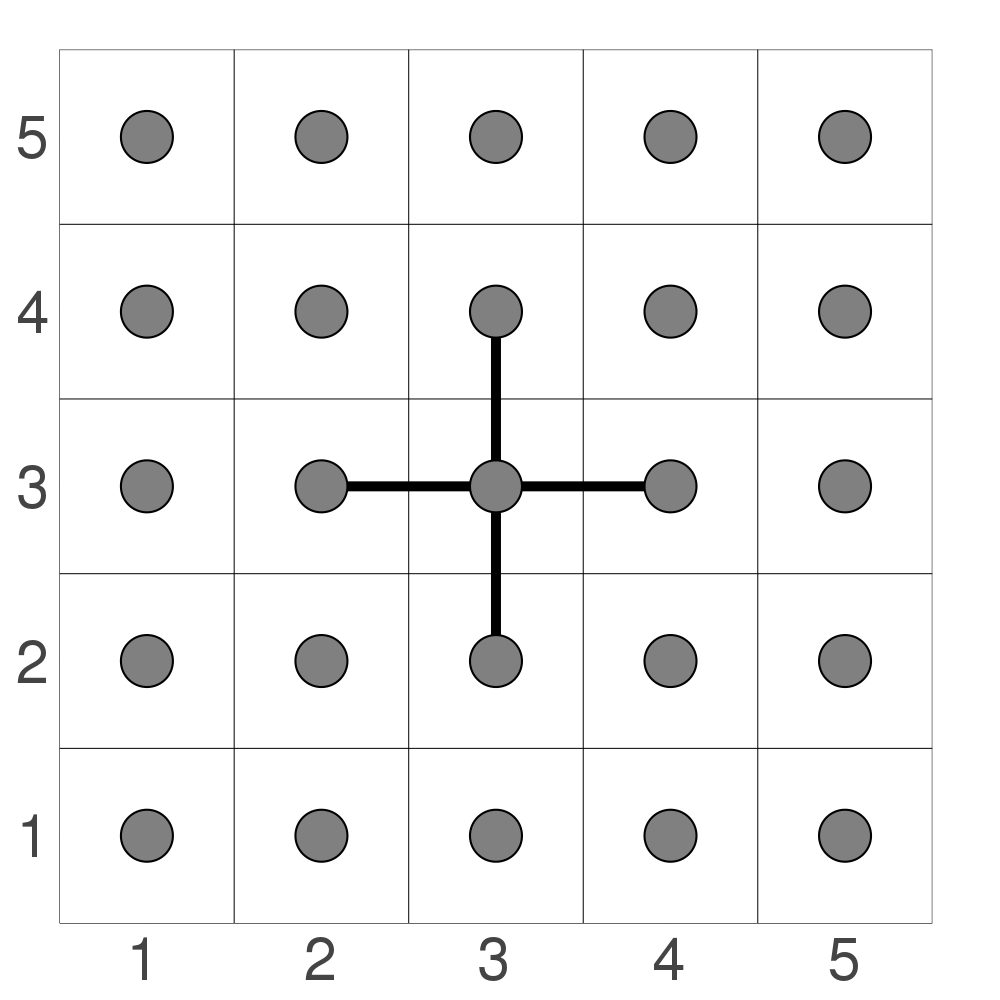Now let's imagine we travel east first. It then enqueues three more nodes: north, south, and east again. This is shown below: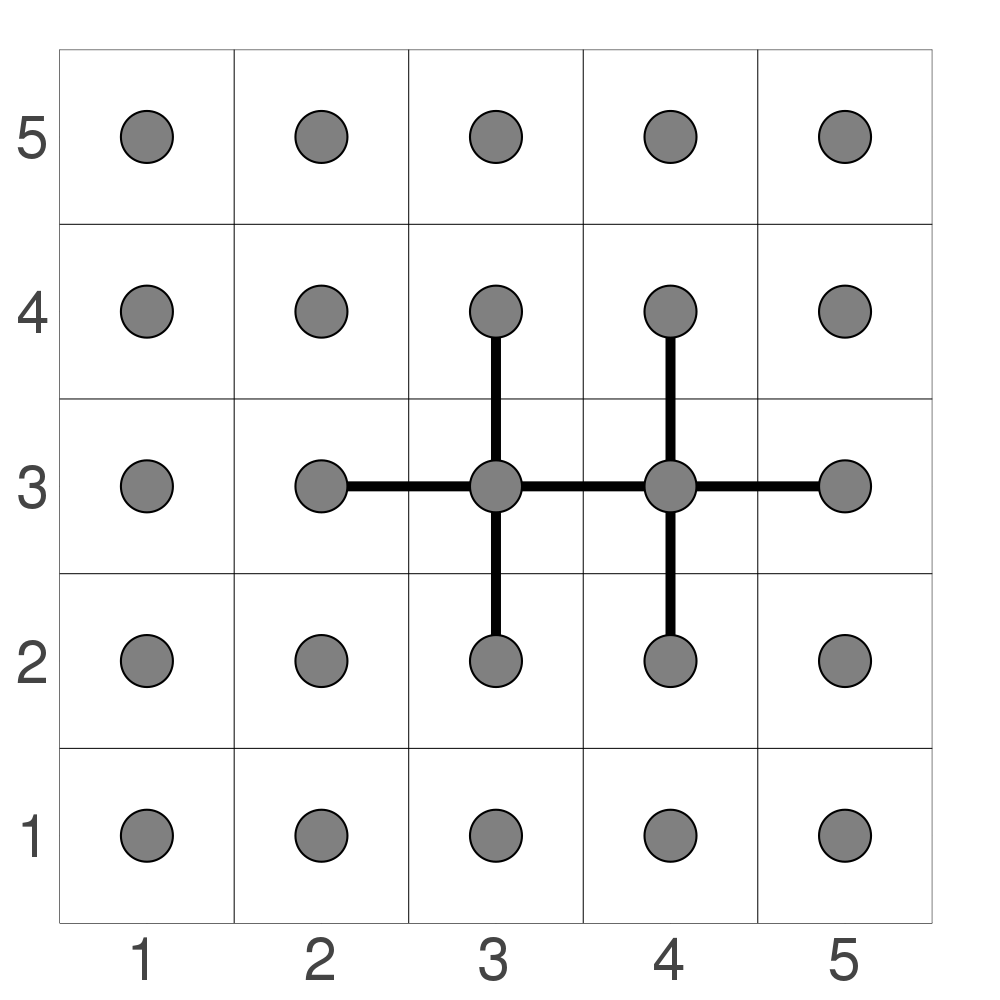It does not enqueue its west neighbor because this has already been colored. At this stage, we will have six nodes ready to be colored and 2 that are already colored. Now let's say we travel north next. This node will enqueue three more nodes: west, north, and east, as shown below: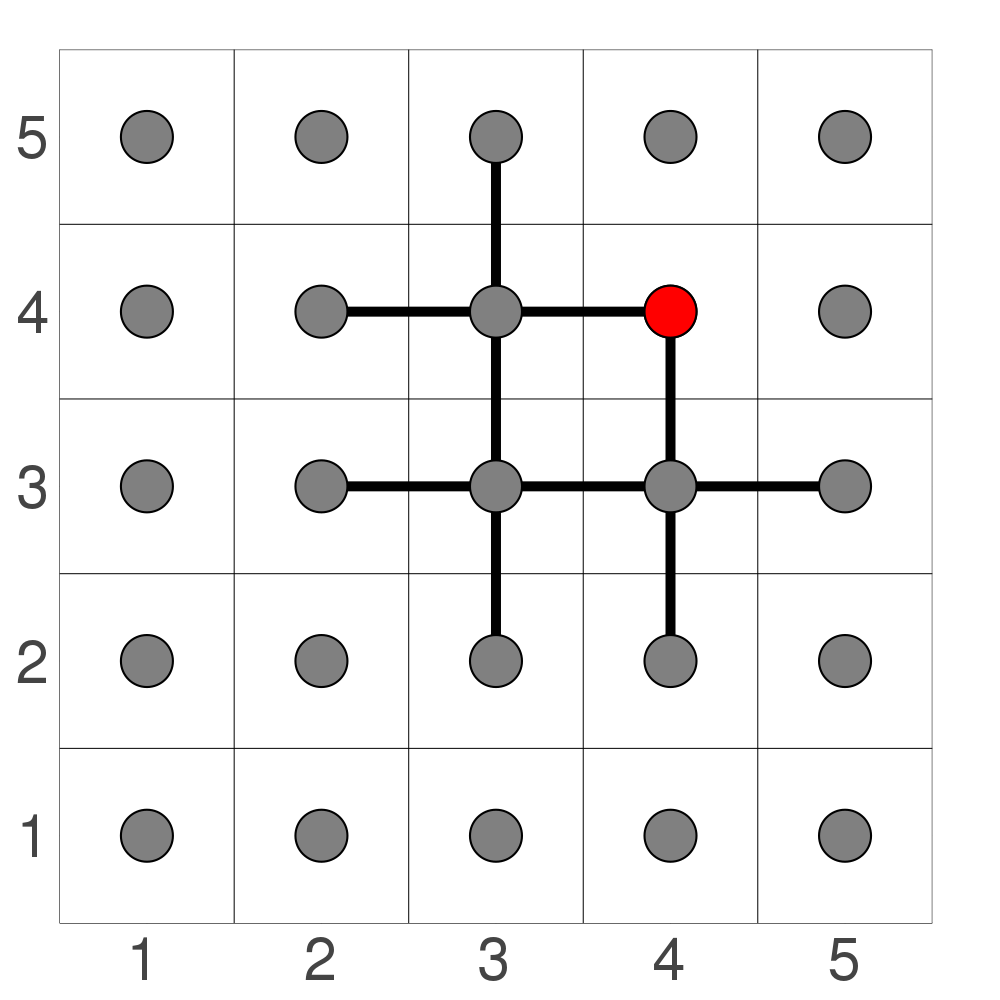The problem is that the east element has already been enqueued for coloring by the previous node!. This shared element is colored in red. As we progress through all four initial neighbors, we will find 4 nodes that are doubly enqueued: all directions diagonal to the initial location! This is again shown below: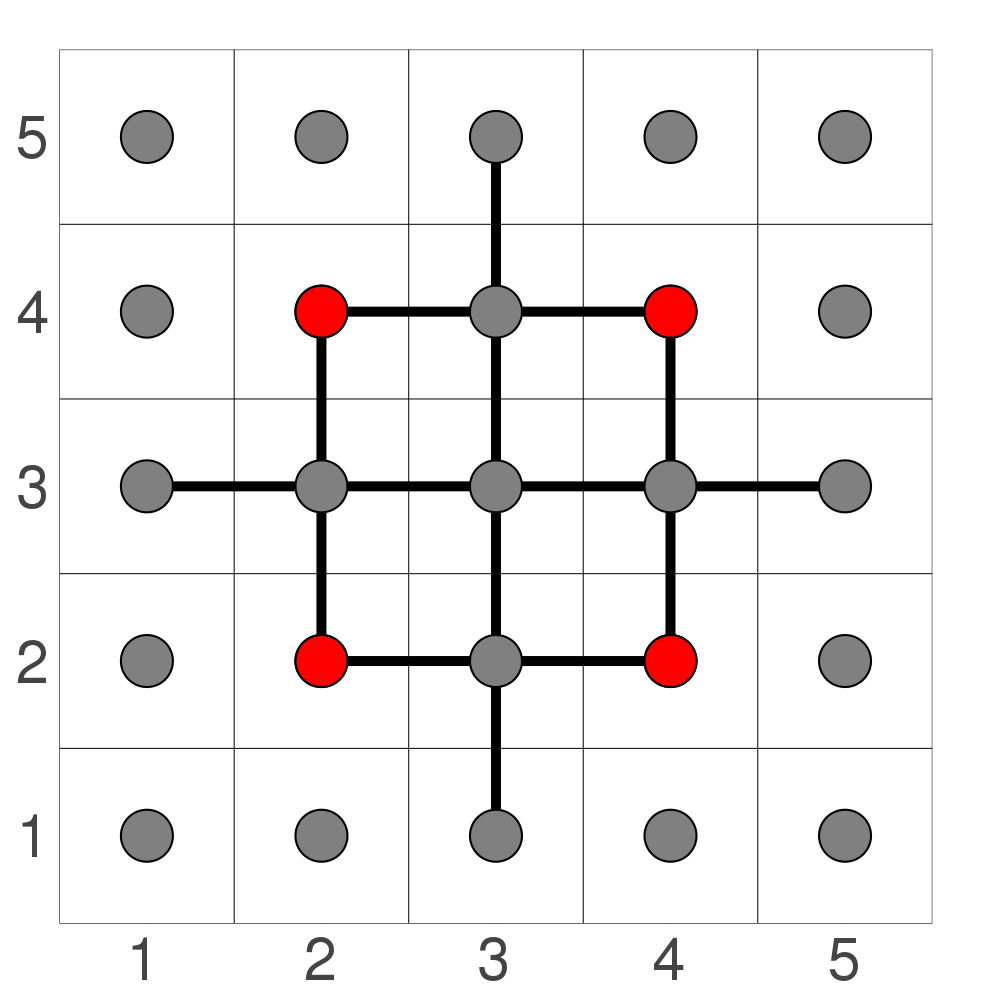As the number of nodes increases, so does the number of duplicate nodes. A quick fix is to color the nodes when they are being enqueued like in the example code above. When doing this, duplicates will not be enqueued with a breadth-first scheme because they will already be colored when other nodes are trying to find their neighbors. This created a node connection pattern like so: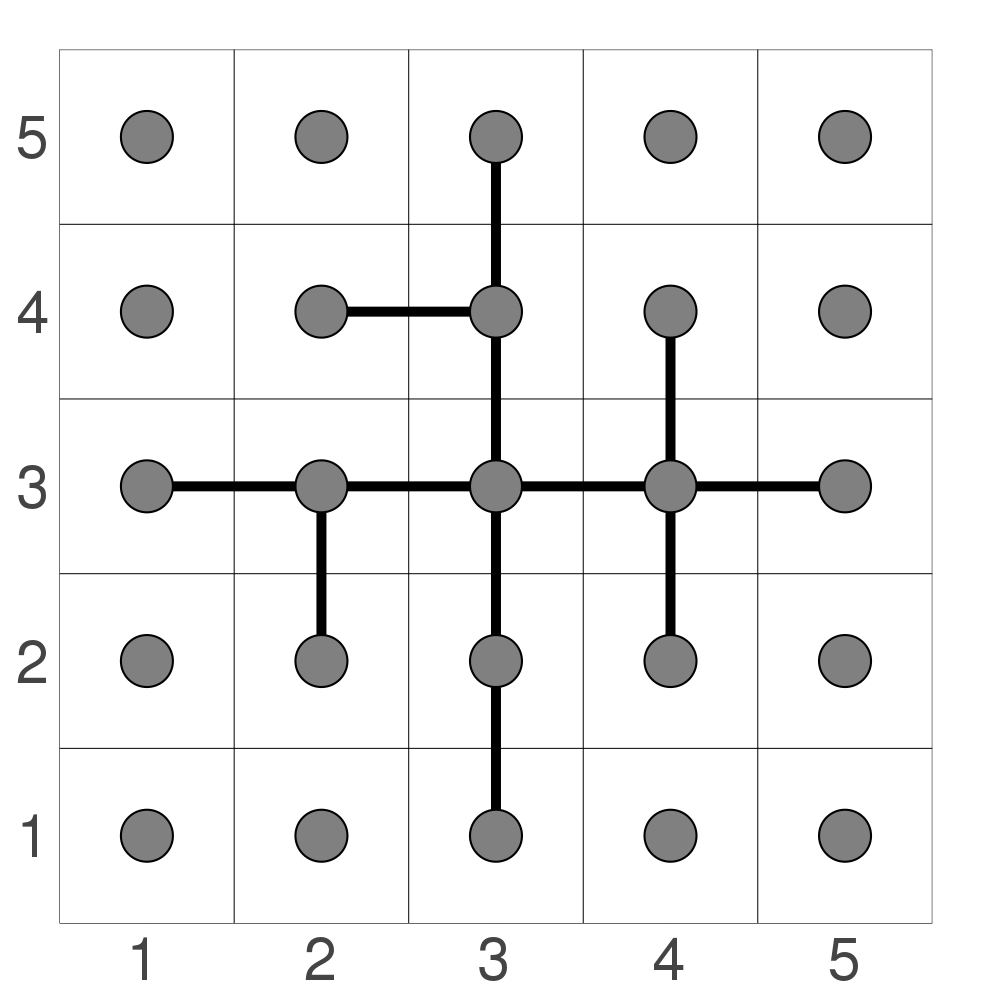As some final food for thought: why wasn't this a problem with the depth-first strategy? The simple answer is that it actually was an issue, but it was way less prevalent. With the depth-first strategy, a number of unnecessary nodes are still pushed to the stack, but because we consistently push one direction before spreading out to other directions, it is more likely that the nodes have filled neighbors when they are looking for what to fill around them.

Simply put: depth-first traversal is slightly more efficient in this case unless you can color as querying for neighbors, in which case breadth-first is more efficient.

## Conclusions

As stated before, the method discussed in this chapter is just the tip of the iceberg and many other flood fill methods exist that are likely to be more efficient for most purposes. These will all be covered in subsequent chapters which will come out somewhat regularly throughout the next few months, lest we flood that archive with flood fill methods.

## Video Explanation

Here is a video describing tree traversal:

## Example Code

The example code for this chapter will be the simplest application of flood fill that still adequately tests the code to ensure it is stopping at boundaries appropriately. For this, we will create a two dimensional array of floats, all starting at 0.0, and then set a single vertical line of elements at the center to be 1.0. After, we will fill in the left-hand side of the array to be all ones by choosing any point within the left domain to fill.

using DataStructures
using Test

# Function to check to make sure we are on the canvas
function inbounds(canvas_size, loc)

# Make sure we are not beneath or to the left of the canvas
if minimum(Tuple(loc)) < 1
return false

# Make sure we are not to the right of the canvas
elseif loc > canvas_size
return false

# Make sure we are not above the canvas
elseif loc > canvas_size
return false
else
return true
end
end

function find_neighbors(canvas, loc::CartesianIndex, old_val, new_val)

# Finding north, south, east, west neighbors
possible_neighbors = [loc + CartesianIndex(0, 1),
loc + CartesianIndex(1, 0),
loc + CartesianIndex(0, -1),
loc + CartesianIndex(-1, 0)]

# Exclusing neighbors that should not be colored
neighbors =  []
for possible_neighbor in possible_neighbors
if inbounds(size(canvas), possible_neighbor) &&
canvas[possible_neighbor] == old_val
push!(neighbors, possible_neighbor)
end
end

return neighbors
end

function stack_fill!(canvas, loc::CartesianIndex, old_val, new_val)
if new_val == old_val
return
end

s = Stack{CartesianIndex}()
push!(s, loc)

while length(s) > 0
current_loc = pop!(s)
if canvas[current_loc] == old_val
canvas[current_loc] = new_val
possible_neighbors = find_neighbors(canvas, current_loc,
old_val, new_val)
for neighbor in possible_neighbors
push!(s,neighbor)
end
end

end
end

function queue_fill!(canvas, loc::CartesianIndex, old_val, new_val)
if new_val == old_val
return
end

q = Queue{CartesianIndex}()
enqueue!(q, loc)

# Coloring the initial location
canvas[loc] = new_val

while length(q) > 0
current_loc = dequeue!(q)

possible_neighbors = find_neighbors(canvas, current_loc,
old_val, new_val)

# Coloring as we are enqueuing neighbors
for neighbor in possible_neighbors
canvas[neighbor] = new_val
enqueue!(q,neighbor)
end

end
end

function recursive_fill!(canvas, loc::CartesianIndex, old_val, new_val)

if (old_val == new_val)
return
end

canvas[loc] = new_val

possible_neighbors = find_neighbors(canvas, loc, old_val, new_val)
for possible_neighbor in possible_neighbors
recursive_fill!(canvas, possible_neighbor, old_val, new_val)
end
end

function main()

# Creation of a 5x5 grid with a single row of 1.0 elements
grid = zeros(5,5)
grid[3,:] .= 1

# Create solution grid

# Start filling at 1,1
start_loc = CartesianIndex(1,1)

@testset "Fill Methods" begin
# Use recursive method and reinitialize grid
recursive_fill!(grid, start_loc, 0.0, 1.0)

grid[1:2,:] .= 0

# Use queue method and reinitialize grid
queue_fill!(grid, start_loc, 0.0, 1.0)

grid[1:2,:] .= 0

# Use stack method and reinitialize grid
stack_fill!(grid, start_loc, 0.0, 1.0)
end

end

main()

#include <stdio.h>
#include <stdlib.h>
#include <string.h>

struct canvas {
int max_x, max_y;
int *data;
};

struct point {
int x, y;
};

struct stack {
size_t top, capacity;
struct point *data;
};

struct queue {
size_t front, back, capacity;
struct point *data;
};

int inbounds(struct point p, struct canvas c) {
return (p.x < 0 || p.y < 0 || p.y >= c.max_y || p.x >= c.max_x) ? 0 : 1;
}

int find_neighbors(struct canvas c, struct point p, int old_val,
struct point *neighbors) {
int cnt = 0;
struct point points = {
{p.x, p.y + 1},
{p.x + 1, p.y},
{p.x, p.y - 1},
{p.x - 1, p.y}
};

for (int i = 0; i < 4; ++i) {
if (inbounds(points[i], c) &&
c.data[points[i].x + c.max_x * points[i].y] == old_val) {
neighbors[cnt++] = points[i];
}
}

return cnt;
}

struct stack get_stack() {
struct stack stk;

stk.data = malloc(4 * sizeof(struct point));
stk.capacity = 4;
stk.top = 0;

return stk;
}

int stack_empty(struct stack stk) {
return stk.top == 0;
}

void stack_push(struct stack *stk, struct point element) {
if (stk->top == stk->capacity) {
stk->capacity *= 2;
stk->data = realloc(stk->data, stk->capacity * sizeof(stk->data));
}

stk->data[stk->top++] = element;
}

struct point stack_pop(struct stack *stk) {
return stk->data[--stk->top];
}

void free_stack(struct stack stk) {
free(stk.data);
}

void stack_fill(struct canvas c, struct point p, int old_val, int new_val) {
if (old_val == new_val) {
return;
}

struct stack stk = get_stack();
stack_push(&stk, p);

while (!stack_empty(stk)) {
struct point cur_loc = stack_pop(&stk);
if (c.data[cur_loc.x + c.max_x * cur_loc.y] == old_val) {
c.data[cur_loc.x + c.max_x * cur_loc.y] = new_val;

struct point neighbors;
int cnt = find_neighbors(c, cur_loc, old_val, neighbors);

for (int i = 0; i < cnt; ++i) {
stack_push(&stk, neighbors[i]);
}
}
}

free_stack(stk);
}

struct queue get_queue() {
struct queue q;

q.data = calloc(4, sizeof(struct point));
q.front = 0;
q.back = 0;
q.capacity = 4;

return q;
}

int queue_empty(struct queue q) {
return q.front == q.back;
}

void enqueue(struct queue *q, struct point element) {
if (q->front == (q->back + 1) % q->capacity) {
size_t size = sizeof(q->data);
struct point *tmp = calloc((q->capacity * 2), size);
memcpy(tmp, q->data + q->front, (q->capacity - q->front) * size);
memcpy(tmp + q->capacity - q->front, q->data, (q->front - 1) * size);

free(q->data);

q->data = tmp;
q->back = q->capacity - 1;
q->front = 0;
q->capacity *= 2;
}

q->data[q->back] = element;
q->back = (q->back + 1) % q->capacity;
}

struct point dequeue(struct queue *q) {
struct point ret = q->data[q->front];
q->front = (q->front + 1) % q->capacity;

return ret;
}

void free_queue(struct queue q) {
free(q.data);
}

void queue_fill(struct canvas c, struct point p, int old_val, int new_val) {
if (old_val == new_val) {
return;
}

struct queue q = get_queue(sizeof(struct point *));
enqueue(&q, p);

while (!queue_empty(q)) {
struct point cur_loc = dequeue(&q);
if (c.data[cur_loc.x + c.max_x * cur_loc.y] == old_val) {
c.data[cur_loc.x + c.max_x * cur_loc.y] = new_val;

struct point neighbors;
int cnt = find_neighbors(c, cur_loc, old_val, neighbors);

for (int i = 0; i < cnt; ++i) {
enqueue(&q, neighbors[i]);
}
}
}

free_queue(q);
}

void recursive_fill(struct canvas c, struct point p, int old_val,
int new_val) {

if (old_val == new_val) {
return;
}

c.data[p.x + c.max_x * p.y] = new_val;

struct point neighbors;
int cnt = find_neighbors(c, p, old_val, neighbors);

for (int i = 0; i < cnt; ++i) {
recursive_fill(c, neighbors[i], old_val, new_val);
}
}

int grid_cmp(int *a, int *b, int size) {
for (int i = 0; i < size; ++i) {
if (a[i] != b[i]) {
return 0;
}
}

return 1;
}

int main() {
int grid = {
0, 0, 0, 0, 0,
0, 0, 0, 0, 0,
1, 1, 1, 1, 1,
0, 0, 0, 0, 0,
0, 0, 0, 0, 0
};
int grid1 = {
0, 0, 0, 0, 0,
0, 0, 0, 0, 0,
1, 1, 1, 1, 1,
0, 0, 0, 0, 0,
0, 0, 0, 0, 0
};
int grid2 = {
0, 0, 0, 0, 0,
0, 0, 0, 0, 0,
1, 1, 1, 1, 1,
0, 0, 0, 0, 0,
0, 0, 0, 0, 0
};
1, 1, 1, 1, 1,
1, 1, 1, 1, 1,
1, 1, 1, 1, 1,
0, 0, 0, 0, 0,
0, 0, 0, 0, 0
};

struct canvas c = {5, 5, grid};
struct canvas c1 = {5, 5, grid1};
struct canvas c2 = {5, 5, grid2};

struct point start_loc = {0, 0};

int pass_cnt = 0;

recursive_fill(c, start_loc, 0, 1);

stack_fill(c1, start_loc, 0, 1);

queue_fill(c2, start_loc, 0, 1);

printf("Test Summary: | Pass\tTotal\n");
printf("Fill Methods  |\t%d\t3\n", pass_cnt);

return 0;
}

#include <array>
#include <cassert>
#include <iostream>
#include <queue>
#include <stack>
#include <vector>

using CartesianIndex = std::array<int, 2>;

auto inbounds(CartesianIndex size, CartesianIndex loc) {
if (loc < 0 || loc < 0) {
return false;
} else if (loc >= size || loc >= size) {
return false;
}
return true;
}

auto find_neighbors(
std::vector<std::vector<float>> const& grid,
CartesianIndex loc,
float old_value,
float /* new_value */) {

const std::vector<CartesianIndex> possible_neighbors{
{loc, loc + 1},
{loc + 1, loc},
{loc, loc - 1},
{loc - 1, loc}};

std::vector<CartesianIndex> neighbors;

for (auto const& possible_neighbor : possible_neighbors) {
const auto size = CartesianIndex{
static_cast<int>(grid.size()), static_cast<int>(grid.size())};
const auto x = static_cast<std::size_t>(possible_neighbor);
const auto y = static_cast<std::size_t>(possible_neighbor);
if (inbounds(size, possible_neighbor) && grid[x][y] == old_value) {
neighbors.push_back(possible_neighbor);
}
}

return neighbors;
}

void recursive_fill(
std::vector<std::vector<float>>& grid,
CartesianIndex loc,
float old_value,
float new_value) {
if (old_value == new_value) {
return;
}

const auto x = static_cast<std::size_t>(loc);
const auto y = static_cast<std::size_t>(loc);

grid[x][y] = new_value;

const auto possible_neighbors = find_neighbors(grid, loc, old_value, new_value);
for (auto const& possible_neighbor : possible_neighbors) {
recursive_fill(grid, possible_neighbor, old_value, new_value);
}
}

void queue_fill(
std::vector<std::vector<float>>& grid,
CartesianIndex loc,
float old_value,
float new_value) {
if (old_value == new_value) {
return;
}

auto q = std::queue<CartesianIndex>{};
q.push(loc);
const auto x = static_cast<std::size_t>(loc);
const auto y = static_cast<std::size_t>(loc);
grid[x][y] = new_value;

while (q.size() > 0) {
const auto current_loc = q.front();
q.pop();
const auto possible_neighbors =
find_neighbors(grid, current_loc, old_value, new_value);
for (auto const& neighbor : possible_neighbors) {
const auto neighbor_x = static_cast<std::size_t>(neighbor);
const auto neighbor_y = static_cast<std::size_t>(neighbor);
grid[neighbor_x][neighbor_y] = new_value;
q.push(neighbor);
}
}
}

void stack_fill(
std::vector<std::vector<float>>& grid,
CartesianIndex loc,
float old_value,
float new_value) {
if (old_value == new_value) {
return;
}

auto s = std::stack<CartesianIndex>{};
s.push(loc);

while (s.size() > 0) {
const auto current_loc = s.top();
s.pop();

const auto x = static_cast<std::size_t>(current_loc);
const auto y = static_cast<std::size_t>(current_loc);

if (grid[x][y] == old_value) {
grid[x][y] = new_value;
const auto possible_neighbors =
find_neighbors(grid, current_loc, old_value, new_value);
for (auto const& neighbor : possible_neighbors) {
s.push(neighbor);
}
}
}
}

int main() {

const std::vector<std::vector<float>> grid{
{0, 0, 1, 0, 0},
{0, 0, 1, 0, 0},
{0, 0, 1, 0, 0},
{0, 0, 1, 0, 0},
{0, 0, 1, 0, 0}};

const std::vector<std::vector<float>> solution_grid{
{1, 1, 1, 0, 0},
{1, 1, 1, 0, 0},
{1, 1, 1, 0, 0},
{1, 1, 1, 0, 0},
{1, 1, 1, 0, 0}};

const CartesianIndex start_loc{1, 1};

auto test_grid = grid;
recursive_fill(test_grid, start_loc, 0.0, 1.0);
assert(test_grid == solution_grid);

test_grid = grid;
queue_fill(test_grid, start_loc, 0.0, 1.0);
assert(test_grid == solution_grid);

test_grid = grid;
stack_fill(test_grid, start_loc, 0.0, 1.0);
assert(test_grid == solution_grid);

return EXIT_SUCCESS;
}

from collections import namedtuple
from queue import Queue
import numpy as np

Point = namedtuple("Point", "x y")

def inbounds(canvas_shape, p):
return min(p) >= 0 and p.x < canvas_shape and p.y < canvas_shape

def find_neighbors(canvas, p, old_val, new_val):
# north, south, east, west neighbors
possible_neighbors = [
Point(p.x, p.y+1),
Point(p.x+1, p.y),
Point(p.x-1, p.y),
Point(p.x, p.y-1)
]

# exclude the neighbors that go out of bounds and should not be colored
neighbors = []
for possible_neighbor in possible_neighbors:
if inbounds(canvas.shape, possible_neighbor):
if canvas[possible_neighbor] == old_val:
neighbors.append(possible_neighbor)
return neighbors

def stack_fill(canvas, p, old_val, new_val):
if old_val == new_val:
return

stack = [p]

while stack:
cur_loc = stack.pop()
canvas[cur_loc] = new_val
stack += find_neighbors(canvas, cur_loc, old_val, new_val)

def queue_fill(canvas, p, old_val, new_val):
if old_val == new_val:
return

q = Queue()
q.put(p)

canvas[p] = new_val

while not q.empty():
cur_loc = q.get()
neighbors = find_neighbors(canvas, cur_loc, old_val, new_val)

for neighbor in neighbors:
canvas[neighbor] = new_val
q.put(neighbor)

def recursive_fill(canvas, p, old_val, new_val):
if old_val == new_val:
return

canvas[p] = new_val

neighbors = find_neighbors(canvas, p, old_val, new_val)
for neighbor in neighbors:
recursive_fill(canvas, neighbor, old_val, new_val)

def main():
grid = np.zeros((5, 5))
grid[2,:] = 1

c0 = grid.copy()
c1 = grid.copy()
c2 = grid.copy()

start_loc = Point(0, 0)

recursive_fill(c0, start_loc, 0, 1)
queue_fill(c1, start_loc, 0, 1)
stack_fill(c2, start_loc, 0, 1)

print("Tests Passed")

if __name__ == "__main__":
main()

from collections import deque
import numpy as np

data Point(x, y):
def __add__(self, other is Point) = Point(self.x + other.x, self.y + other.y)

# This function is necessary, because negative indices wrap around the
# array in Coconut.
def inbounds(canvas_shape, location is Point) =
min(location) >= 0 and location.x < canvas_shape and location.y < canvas_shape

def find_neighbours(canvas, location is Point, old_value):
possible_neighbours = ((Point(0, 1), Point(1, 0), Point(0, -1), Point(-1, 0))
|> map$(location.__add__)) yield from possible_neighbours |> filter$(x -> (inbounds(canvas.shape, x)
and canvas[x] == old_value))

def stack_fill(canvas, location is Point, old_value, new_value):
if new_value == old_value or not inbounds(canvas.shape, location):
return

stack = [location]

while stack:
current_location = stack.pop()
if canvas[current_location] == old_value:
canvas[current_location] = new_value
stack.extend(find_neighbours(canvas, current_location, old_value))

def queue_fill(canvas, location is Point, old_value, new_value):
if new_value == old_value or not inbounds(canvas.shape, location):
return

queue = deque()
queue.append(location)

canvas[location] = new_value

while queue:
current_location = queue.popleft()
for neighbour in find_neighbours(canvas, current_location, old_value):
canvas[neighbour] = new_value
queue.append(neighbour)

def recursive_fill(canvas, location is Point, old_value, new_value):
if new_value == old_value or not inbounds(canvas.shape, location):
return

canvas[location] = new_value
# consume is important here, because otherwise, the recursive function is not called again
consume(
find_neighbours(canvas, location, old_value)
|> map$(recursive_fill$(canvas, ?, old_value, new_value))
)

def test_grid(initial_canvas, final_canvas, function):
canvas = initial_canvas.copy() # ensure the initial_canvas is unchanged
function(canvas)
return (canvas == final_canvas).all()

def test():
from collections import namedtuple

TestResults = namedtuple('TestResults', 'passes failures')
pass_count = failure_count = 0

grid = np.zeros((5, 5))
grid[2,:] = 1
solution_grid = np.zeros((5, 5))
solution_grid[:3,] = 1

starting_location = Point(0, 0)

recursive_test_func = recursive_fill$(?, starting_location, 0, 1) # The following is manual unit testing of the function if test_grid(grid, solution_grid, recursive_test_func): pass_count += 1 print('.', end='') else: failure_count += 1 print('F', end='') stack_test_func = stack_fill$(?, starting_location, 0, 1)
if test_grid(grid, solution_grid, stack_test_func):
print('.', end='')
pass_count += 1
else:
print('F', end='')
failure_count += 1

queue_test_func = queue_fill\$(?, starting_location, 0, 1)
if test_grid(grid, solution_grid, queue_test_func):
print('.', end='')
pass_count += 1
else:
print('F', end='')
failure_count += 1

print()
print(TestResults(pass_count, failure_count))

if __name__ == '__main__':
# Testing setup
test()


### Bibliography

2.
Torbert, Shane, Applied computer science, Springer, 2016.

##### Code Examples